• This event has passed.

# Title: Factorization of positive definite functions through convolution and the Turàn problem

Abstract: If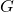is a finite abelian group, we call a subset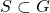symmetric if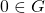and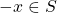whenever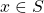. We also let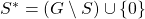. We consider the problem of expressing an arbitrary positive definite function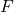onas the convolution product of two positive definite functions, one supported on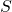and the other one supported on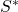. We show that, in the particular case whereis the constant function, this problem is related to the Tur\’an problem for positive definite functions. In the particular case of a finite abelian group, this last problem asks the following question. Given a symmetric set, find the maximum value of the sum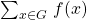if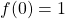and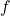is a positive definite function onsupported on. We introduce the notion of  {\it dual Tur\’an problem for}, which is essentially the Tur\’an problem for the set, and show how the Tur\’an problem forand its dual are related, and how the factorization mentioned above plays a role is solving both those problems. We will then give an overview of how these results can be extended to other abelian groups such as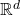.

The Dalhousie-AARMS Analysis-Applied Math-Physics Seminar takes place on Fridays from 4 – 5 pm Atlantic Time over Zoom.  If you would like to attend, please email the organizers for connection details.

## Details

Date:
October 30, 2020
Time:
4:00 pm - 5:00 pm
Event Category:
Website:
https://sureshes.wordpress.com/dalhousie-analysis-seminar/

Zoom seminar

## Organizer

Suresh Eswarathasan
Email:
sr766936@dal.ca